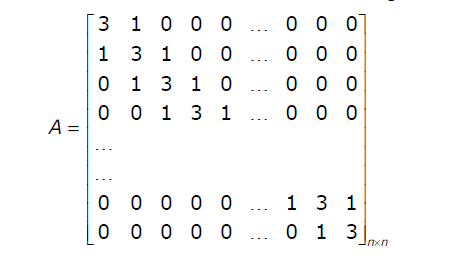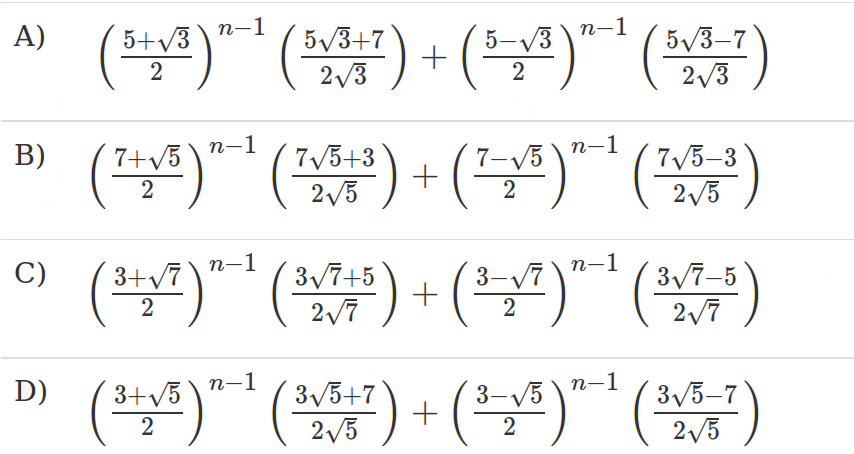# GATE | GATE-IT-2004 | Question 32

• Last Updated : 28 Jun, 2021

Let A be an Let A be an n × n matrix of the following form.Attention reader! Don’t stop learning now.  Practice GATE exam well before the actual exam with the subject-wise and overall quizzes available in GATE Test Series Course.

Learn all GATE CS concepts with Free Live Classes on our youtube channel.

What is the value of the determinant of A?(A) A
(B) B
(C) C
(D) D

Explanation:

```The first thing you need to get by seeing these type of questions is:-
Go for substitution method.
For n=2, the values will be
A) 16
B) 26
C) 7
D) 8
As all the values are unique for a small value of n, it does not take much time.
The given matrix will be A = [3 1]
[1 3]
So, det(A) = 3*3-1*1 = 8
```
```   Alternative method:-
You can frame the relations in between det(An+1), det(An), det(An-1)
i.e. d(An+1) = 3*d(An) - d(An-1)
X   = 3*X^0 - X^-1
X^2 = 3*x - 1
Solution for this equation is (3+sqrt(5))/2, (3-sqrt(5))/2
The only option which has roots of type (3+sqrt(5)) is D.
From this, you can match the options easily.
```

This explanation has been provided by Anil Saikrishna.

Quiz of this Question

My Personal Notes arrow_drop_up